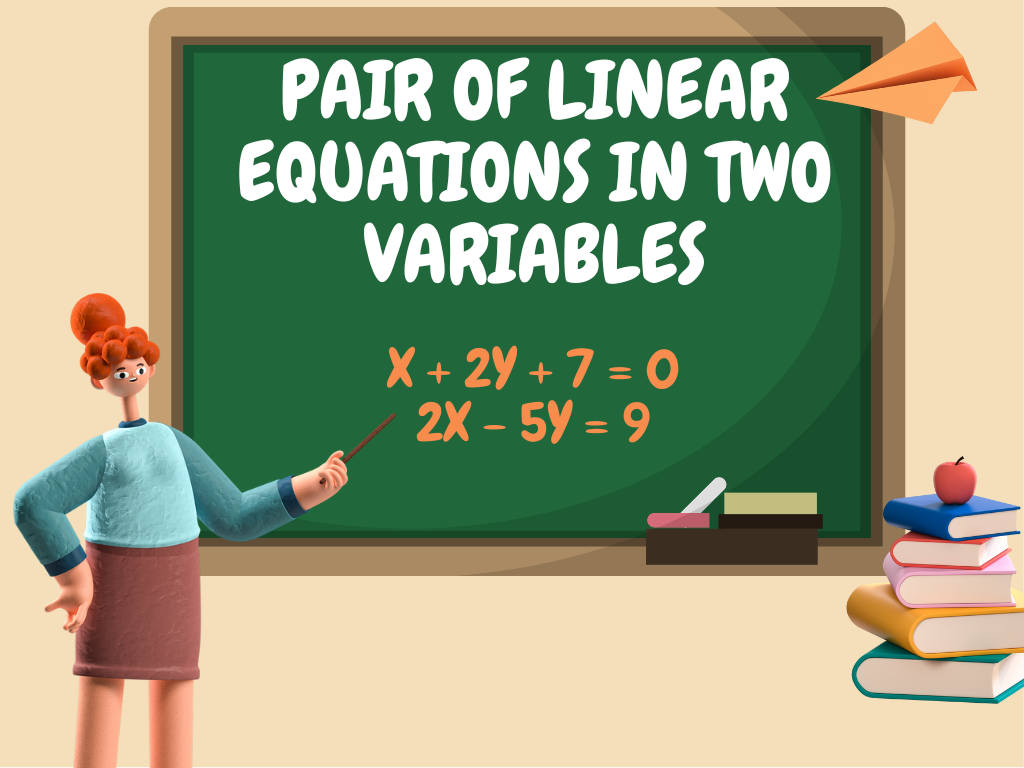## Pair of Linear Equations in Two Variables Class 10th

We are familiar with linear equations in two variables as we have studied them in previous classes. The linear equation having two variables is called the linear equation in two variables. In class 10th, we shall study the pair of linear equations in two variables, the solution of a pair of linear equations in two …